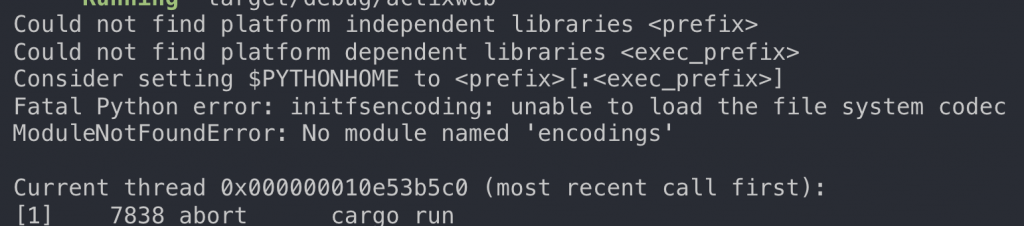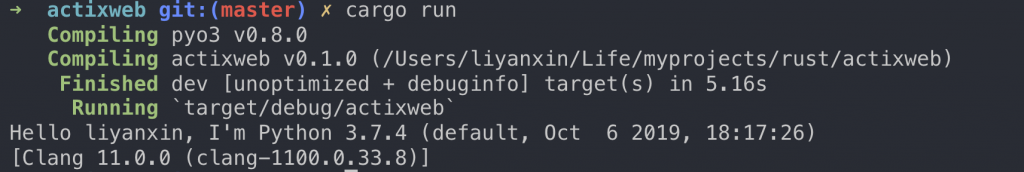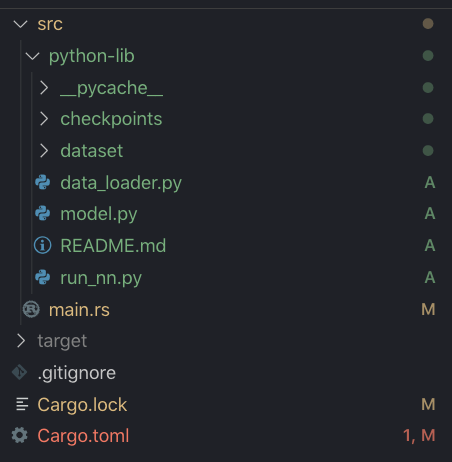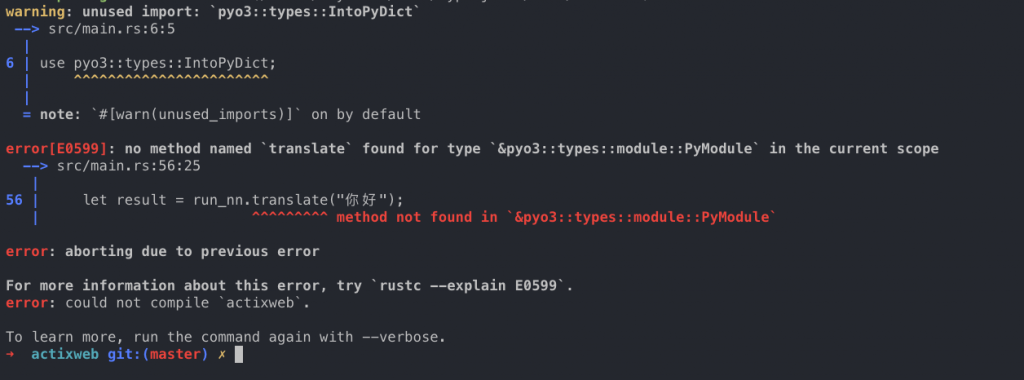#DAY 20
0

## FFI(Foreign Function Interface)

``````extern "C" {
fn abs(input: i32) -> i32;
}

fn main() {
unsafe {
println!("Absolute value of -3 according to C: {}", abs(-3));
}
}
``````

## PyO3

``````\$ rustup toolchain install nightly
\$ rustup default nightly
``````

``````[dependencies]
pyo3 = "0.8.0"
``````

``````use pyo3::prelude::*;
use pyo3::types::IntoPyDict;

fn main() -> PyResult<()> {
let gil = Python::acquire_gil();
let py = gil.python();
let sys = py.import("sys")?;
let version: String = sys.get("version")?.extract()?;

let locals = [("os", py.import("os")?)].into_py_dict(py);
let code = "os.getenv('USER') or os.getenv('USERNAME') or 'Unknown'";
let user: String = py.eval(code, None, Some(&locals))?.extract()?;

println!("Hello {}, I'm Python {}", user, version);
Ok(())
}
``````]

``````def translate(original_sentence):
# preprocessing the original_sentence
init_jieba_dict()
sentence = seg2words(original_sentence)
sentence = preprocess_ch_sentence(sentence)

...
``````

``````if __name__ == '__main__':

# if len(sys.argv) != 2 or sys.argv not in ['train','translate']:
#     raise ValueError("""usage: python run_nn.py [train/translate]""")

if sys.argv == 'train':
train()

if sys.argv == 'translate':
translate()
``````

``````fn main() -> PyResult<()> {
let gil = Python::acquire_gil();
let py = gil.python();
let run_nn = py.import("./python-lib/run_nn")?;
let result = run_nn.translate("你好");
// let sys = py.import("sys")?;
// let version: String = sys.get("version")?.extract()?;
// let locals = [("os", py.import("os")?)].into_py_dict(py);
// let code = "os.getenv('USER') or os.getenv('USERNAME') or 'Unknown'";
// let user: String = py.eval(code, None, Some(&locals))?.extract()?;
println!("{}", result);
Ok(())
}
``````## 最後一樣有問題歡迎發問## 參考網站

Foreign,Function,Interface

python_from_rust